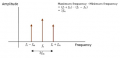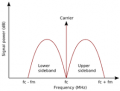# AM Modulation Question. Are sidebands created without a mixer?

#### Beetle_X

Joined Nov 2, 2012
52
The only info I had until recently is with AM, the audio freqeuncy is superimposed on the carrier wave. I figured that the amplitude of a single frequency was varied and that was it. Now I read that side bands are always produced but not all AM modulators have a mixer. Simple AM modulators only have a oscillator ,a transformer , mic , battery and a antenna. I doubt that this set up can create side bands. Would someone please point me in the right direction on this?

#### Beetle_X

Joined Nov 2, 2012
52
If we use this guide as an example, Diagrams E and F , the O scope represents a carrier wave with it's amplitude manipulated and the Spectrum Analyzer represents side bands which appeared from no where. How do we go from a manipulated carrier to which is a single frequency to multiple frequencies?

#### AlbertHall

Joined Jun 4, 2014
10,783
You either accept that that is what happens or follow through the maths which proves it.

#### AlbertHall

Joined Jun 4, 2014
10,783
I remember when I was learning electronics being very confused about audio and RF mixers. They are both called mixers but they are very different. A good audio mixer is linear (no distortion) and is not supposed to produce sidebands beacause of that. An RF mixer can be just a non-linear circuit (produces distortion) and is intended to produce sidebands.

#### Papabravo

Joined Feb 24, 2006
14,872
I remember when I was learning electronics being very confused about audio and RF mixers. They are both called mixers but they are very different. A good audio mixer is linear (no distortion) and is not supposed to produce sidebands beacause of that. An RF mixer can be just a non-linear circuit (produces distortion) and is intended to produce sidebands.
That is the way I remember it as well. Non-linear process produce new frequencies (aka sidebands). Linear processes, aka addition, do NOT.

•bertus

#### sparky 1

Joined Nov 3, 2018
405
The wave shape in bandwidth concept can be singular such as a pure sine. The modulation index has a wide gradient. With very low modulation the form remains in tact as the AC voltage of modulation increases the singular sine begins to take on more effects from compression and refraction. The shape starts on the outer surfaced. Finally the modulation index is high enough the inward and outward shape divides the wave.

#### MrChips

Joined Oct 2, 2009
22,550
Let us look at the math.

A simple sine wave can be represented as
V = A sin(2πft)
where f is the carrier frequency
We modulate the amplitude A with low frequency sine wave of frequency g. (I use a cosine just to keep the formula simple).

Using the following formula
sin(A).cos(B) = ½ sin(A+B) + ½ sin(A-B)

V = A cos(2πgt) x sin(2πft)
= ½ A sin(2πft + 2πgt) + ½ A sin(2πft - 2πgt)
= ½ A sin(2πt (f+g) ) + ½ A sin(2πt (f-g) )

Besides the carrier frequency f we end up with additional frequencies (f+g) and (f-g) which are the upper and lower sidebands respectively.

It is helpful to look at the frequency spectrum:For a band of modulating frequencies such as voice or music, this is what the frequency spectrum would look like:All the information is in the sideband. Hence SSB (single sideband) transmission removes one of the sidebands and the carrier. Only a single sideband is transmitted thus conserving RF bandwidth and transmitted power.

•Beetle_X